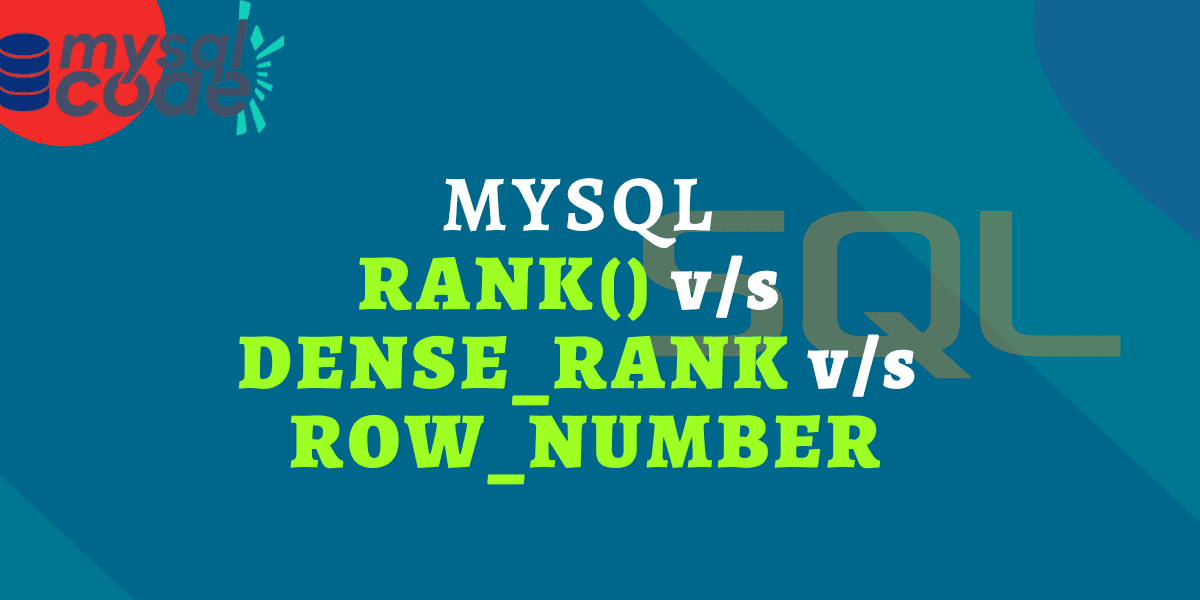# MySQL Rank Function Vs Row Number Vs Dense RankWindow functions in mysql are a little bit confusing and without trying them in the real-time examples, they are even more confusing! The RANK, DENSE_RANK and ROW_NUMBER functions are used to return the row numbers in the table; however, they all work totally differently.

In this tutorial, we will see the overview of all functions with examples to understand how they differ from each other. So, let’s dive into it!

Also read: MySQL Window Functions Vs GROUP BY

## Introduction

The RANK(), DENSE_RANK(), and ROW_NUMBER() are window functions that return the row numbers based on the partition and the ORDER BY clause.

Note that, because all these functions are window functions, they reset every time a new partition occurs. This means the value resets to 1 when the new partition arrives.

Let’s first create a table with some data in it to demonstrate the examples of each function.

Here, we will be using the following table which consists of the student marks.

As you can see here, there is a total of twenty-five students from the five classes. This means we can create a partition of students based on the class. But we will keep it simple and proceed without using the PARTITION BY subclause.

## RANK() Function in MySQL

The RANK() function returns the row number to each row in the table. However, it doesn’t assign consecutive row numbers. For rows having the same value, it assigns the same number. To the next unique row, instead of assigning the +1 of the previous count, it simply assigns the row number from the beginning.

Let’s understand it by example. Suppose there are ten rows in the table. Row numbers 1, 2, and 3 have the same values. Here, the rank function will assign rank 1 to the first three rows because they have the same value. The next row number that will be assigned to row number 4 is 4.

Now we will write a query to assign the rankings to the students according to their marks. We will assign the top ranks to the students having the maximum marks. Therefore, we need to sort the rows in descending order of the marks.

```.wp-block-code {
border: 0;
}

.wp-block-code > span {
display: block;
overflow: auto;
}

.shcb-language {
border: 0;
clip: rect(1px, 1px, 1px, 1px);
-webkit-clip-path: inset(50%);
clip-path: inset(50%);
height: 1px;
margin: -1px;
overflow: hidden;
position: absolute;
width: 1px;
word-wrap: normal;
word-break: normal;
}

.hljs {
box-sizing: border-box;
}

.hljs.shcb-code-table {
display: table;
width: 100%;
}

.hljs.shcb-code-table > .shcb-loc {
color: inherit;
display: table-row;
width: 100%;
}

.hljs.shcb-code-table .shcb-loc > span {
display: table-cell;
}

.wp-block-code code.hljs:not(.shcb-wrap-lines) {
white-space: pre;
}

.wp-block-code code.hljs.shcb-wrap-lines {
white-space: pre-wrap;
}

.hljs.shcb-line-numbers {
border-spacing: 0;
counter-reset: line;
}

.hljs.shcb-line-numbers > .shcb-loc {
counter-increment: line;
}

.hljs.shcb-line-numbers .shcb-loc > span {
}

.hljs.shcb-line-numbers .shcb-loc::before {
border-right: 1px solid #ddd;
content: counter(line);
display: table-cell;
text-align: right;
-webkit-user-select: none;
-moz-user-select: none;
-ms-user-select: none;
user-select: none;
white-space: nowrap;
width: 1%;
}```SELECT *, RANK() OVER(ORDER BY marks DESC) AS 'rank'
FROM marks;```Code language: SQL (Structured Query Language) (sql)```

As you can see here, rank 7 is assigned to four students. Whereas, the next rank assigned is 11 because the rank function doesn’t assign consecutive row numbers. You can read our detailed guide on the RANK() function here.

## DENSE_RANK() Function in MySQL

The DENSE_RANK() function is very similar to the RANK() function. However, instead of skipping the ranks, the DENSE_RANK() function assigns the consecutive ranking numbers to the next rows.

For example,

if the first three rows have the same value then each will be assigned the rank number 1 and the fourth row will get the rank 2. This means consecutive numbers are assigned to the row.

Let’s write a query now to assign the ranks to students using the DENSE_RANK() function.

``````SELECT *, DENSE_RANK()
OVER(ORDER BY marks DESC) AS 'dense_rank'
FROM marks;```Code language: SQL (Structured Query Language) (sql)```

As you can see from the result, consecutive row numbers are assigned to the rows.

You can read our detailed guide on the DENSE_RANK() function here.

## ROW_NUMBER() Function in MySQL

The ROW_NUMBER() function assigns a unique number to each row, no matter how many duplicate values are there. Unlike the RANK and DENSE_RANK functions, the ROW_NUMBER simply ignores the same values of two or more rows and keeps assigning consecutive numbers.

The ROW_NUMBER is the simplest window function to understand to use. It is majorly used when you want to get the first or last record from the table when there is no auto_increment key column.

Let’s see it in the action.

``````SELECT *,
ROW_NUMBER() OVER(ORDER BY marks DESC) as 'row_number'
FROM marks;```Code language: SQL (Structured Query Language) (sql)```

Here you can see, no two rows have the same row number. Each row in the table has a unique and consecutive row number. You can read our detailed guide on the ROW_NUMBER() function here.

## RANK() vs DENSE_RANK() vs ROW_NUMBER()

Let’s first understand the similarities between these three functions.

All these functions return the numbers to each row in the table. However, the method of assigning the numbers to rows is different.

Also, all of them require the ORDER BY subclause inside the OVER clause. Without the ORDER BY subclause, each row will be assigned with the number 1 except for the row_number function.

When you use the PARTITION BY subclause in these functions, the count resets to 1 when a new partition arrives.

Now, let’s use these three functions in a single query to see the output difference.

``````SELECT *,
RANK() OVER(ORDER BY marks DESC) AS 'rank',
DENSE_RANK() OVER(ORDER BY marks DESC) AS 'dense_rank',
ROW_NUMBER() OVER(ORDER BY marks DESC) AS 'row_num'
FROM marks;```Code language: SQL (Structured Query Language) (sql)```